### Criteria for Optimality

PROC NLP solves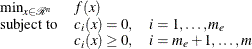where
is the objective function and the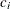’s are the constraint functions.

A pointis feasible if it satisfies all the constraints. The feasible region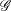is the set of all the feasible points. A feasible point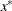is a global solution of the preceding problem if no point inhas a smaller function value than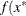). A feasible pointis a local solution of the problem if there exists some open neighborhood surroundingin that no point has a smaller function value than). Nonlinear programming algorithms cannot consistently find global minima. All the algorithms in PROC NLP find a local minimum for this problem. If you need to check whether the obtained solution is a global minimum, you may have to run PROC NLP with different starting points obtained either at random or by selecting a point on a grid that contains.

Every local minimizerof this problem satisfies the following local optimality conditions:

• The gradient (vector of first derivatives)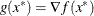of the objective function(projected toward the feasible region if the problem is constrained) at the pointis zero.

• The Hessian (matrix of second derivatives)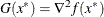of the objective function(projected toward the feasible regionin the constrained case) at the pointis positive definite.

Most of the optimization algorithms in PROC NLP use iterative techniques that result in a sequence of points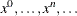, that converges to a local solution. At the solution, PROC NLP performs tests to confirm that the (projected) gradient is close to zero and that the (projected) Hessian matrix is positive definite.

#### Karush-Kuhn-Tucker Conditions

An important tool in the analysis and design of algorithms in constrained optimization is the Lagrangian function, a linear combination of the objective function and the constraints: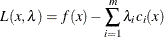The coefficients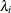are called Lagrange multipliers. This tool makes it possible to state necessary and sufficient conditions for a local minimum. The various algorithms in PROC NLP create sequences of points, each of which is closer than the previous one to satisfying these conditions.

Assuming that the functionsandare twice continuously differentiable, the pointis a local minimum of the nonlinear programming problem, if there exists a vector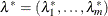that meets the following conditions.

1. First-order Karush-Kuhn-Tucker conditions: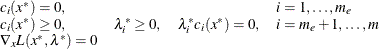2. Second-order conditions: Each nonzero vector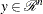that satisfies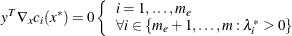also satisfies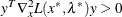Most of the algorithms to solve this problem attempt to find a combination of vectorsandfor which the gradient of the Lagrangian function with respect tois zero.

#### Derivatives

The first- and second-order conditions of optimality are based on first and second derivatives of the objective functionand the constraints.

The gradient vector contains the first derivatives of the objective functionwith respect to the parameters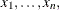as follows: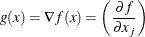Thesymmetric Hessian matrix contains the second derivatives of the objective functionwith respect to the parametersas follows: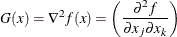For least squares problems, theJacobian matrix contains the first-order derivatives of theobjective functions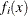with respect to the parametersas follows: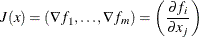In the case of least squares problems, the crossproduct Jacobian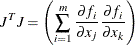is used as an approximate Hessian matrix. It is a very good approximation of the Hessian if the residuals at the solution are small. (If the residuals are not sufficiently small at the solution, this approach may result in slow convergence.) The fact that it is possible to obtain Hessian approximations for this problem that do not require any computation of second derivatives means that least squares algorithms are more efficient than unconstrained optimization algorithms. Using the vector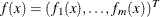of function values, PROC NLP computes the gradient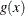by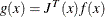TheJacobian matrix contains the first-order derivatives of thenonlinear constraint functions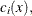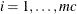, with respect to the parameters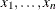, as follows: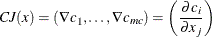PROC NLP provides three ways to compute derivatives:

• It computes analytical first- and second-order derivatives of the objective functionwith respect to thevariables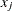.

• It computes first- and second-order finite-difference approximations to the derivatives. For more information, see the section Finite-Difference Approximations of Derivatives.

• The user supplies formulas for analytical or numerical first- and second-order derivatives of the objective function in the GRADIENT, JACOBIAN, CRPJAC, and HESSIAN statements. The JACNLC statement can be used to specify the derivatives for the nonlinear constraints.